# Jacobian

(redirected from Derivative of a multivariable function)
Also found in: Dictionary.
Related to Derivative of a multivariable function: Partial Derivatives

## Jacobian

[jə′kō·bē·ən]
(mathematics)
The Jacobian of functions ƒi (x1, x2, …, xn ), i = 1, 2, …, n, of real variables xi is the determinant of the matrix whose i th row lists all the first-order partial derivatives of the function ƒi (x1, x2, …, xn ). Also known as Jacobian determinant.

## Jacobian

(or functional determinant), a determinant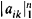with elements aik = ∂yi/∂xk where yi = fi(x1, . . ., xn), 1 ≤ in, are functions that have continuous partial derivatives in some region Δ. It is denoted by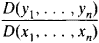The Jacobian was introduced by K. Jacobi in 1833 and 1841. If, for example, n = 2, then the system of functions

(1) yl = f1(x1, x2) y2 = f2(x1, x2)

defines a mapping of a region Δ, which lies in the plane x1x2, onto a region of the plane y1y2. The role of the Jacobian for the mapping is largely analogous to that of the derivative for a function of a single variable. Thus, the absolute value of the Jacobian at some point M is equal to the local factor by which areas at the point are altered by the mapping; that is, it is equal to the limit of the ratio of the area of the image of the neighborhood of M to the area of the neighborhood as the dimensions of the neighborhood approach zero. The Jacobian at M is positive if mapping (1) does not change the orientation in the neighborhood of M, and negative otherwise.

If the Jacobian does not vanish in the region Δ and if φ(y1, y2) is a function defined in the region Δ1 (the image of Δ), then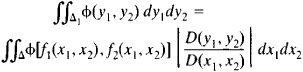(the formula for change of variables in a double integral). An analogous formula obtains for multiple integrals. If the Jacobian of mapping (1) does not vanish in region Δ, then there exists the inverse mapping

x1 = ψ(y1, y2) x2 = ψ2(y1, y2)

and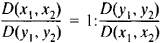(an analogue of the formula for differentiation of an inverse function). This assertion finds numerous applications in the theory of implicit functions.

In order for the explicit expression, in the neighborhood of the point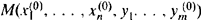, of the functions y1, . . . . ym that are implicitly defined by the equations

(2) Fk (x1. . . .,xn, y1. . .,ym) = 0 Ikm

to be possible, it is sufficient that the coordinates of M satisfy equations (2), that the functions Fk have continuous partial derivatives, and that the Jacobian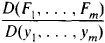be nonzero at M.

### REFERENCES

Kudriavtsev, L. D. Matematicheskii analiz, 2nd ed., vol. 2. Moscow, 1973.
Il’in, V. A., and E. G. Pozniak. Osnovy matematicheskogo analiza, 3rd ed.. part I. Moscow, 1971.
Site: Follow: Share:
Open / Close